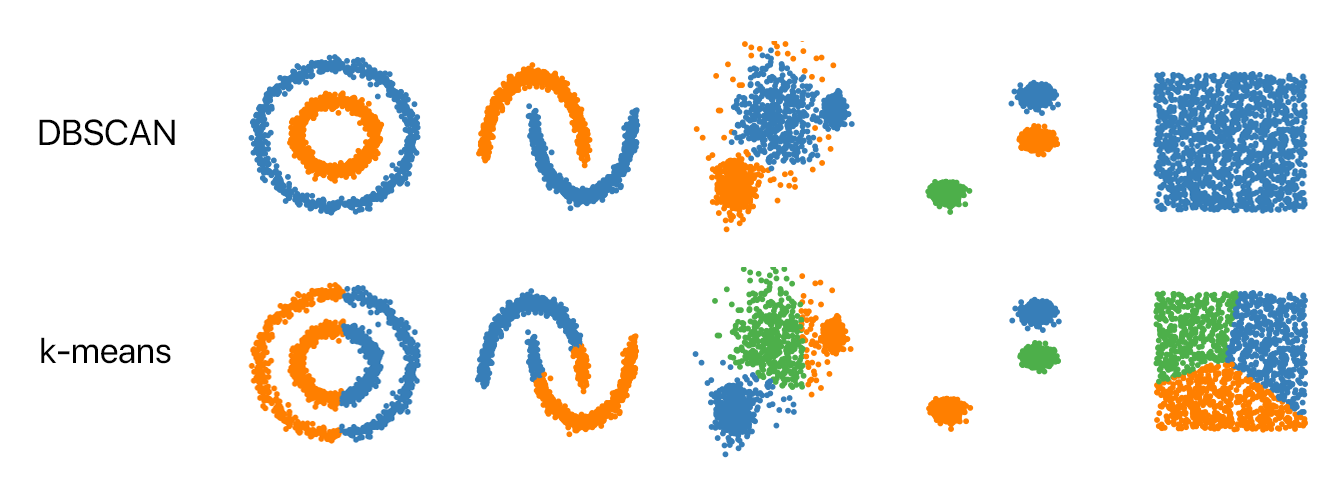Swiftpack.co - Package - NSHipster/DBSCAN

# DBSCANDensity-based spatial clustering of applications with noise.

DBSCAN is a clustering algorithm. Give it a collection of values and the algorithm organizes them into groups of nearby values.

For many of us, if we're familiar with clustering algorithms at all, we know about k-means clustering. But one of the challenges with k-means is that you need to specify a number of clusters ("k") in order to use it. Much of the time, we won't know what a reasonable k value is a priori. (In fact, that's often what we want to know in the first place!)

What's nice about DBSCAN is that you don't have to specify a number of clusters to use it. All you need is a function to calculate distance between values and some guidance for what amount of distance is considered "close". DBSCAN also produces more reasonable results than k-means across a variety of different distributions.## Usage

``````import DBSCAN
import simd

let input: [SIMD3<Double>] = [[ 0, 10, 20 ],
[ 0, 11, 21 ],
[ 0, 12, 20 ],
[ 20, 33, 59 ],
[ 21, 32, 56 ],
[ 59, 77, 101 ],
[ 58, 79, 100 ],
[ 58, 76, 102 ],
[ 300, 70, 20 ],
[ 500, 300, 202],
[ 500, 302, 204 ]]

let dbscan = DBSCAN(input)

#if swift(>=5.2)
let (clusters, outliers) = dbscan(epsilon: 10,
minimumNumberOfPoints: 1,
distanceFunction: simd.distance)
#else // Swift <5.2 requires explicit `callAsFunction` method name
let (clusters, outliers) = dbscan.callAsFunction(epsilon: 10,
minimumNumberOfPoints: 1,
distanceFunction: simd.distance)
#endif

print(clusters)
// [ [0, 10, 20], [0, 11, 21], [0, 12, 20] ]
// [ [20, 33, 59], [21, 32, 56] ],
// [ [58, 79, 100], [58, 76, 102], [59, 77, 101] ],
// [ [500, 300, 202], [500, 302, 204] ],

print(outliers)
// [ [ 300, 70, 20 ] ]
``````

• Swift 5.1+

## Installation

### Swift Package Manager

Add the DBSCAN package to your target dependencies in `Package.swift`:

``````import PackageDescription

let package = Package(
name: "YourProject",
dependencies: [
.package(
url: "https://github.com/NSHipster/DBSCAN",
from: "0.0.1"
),
]
)
``````

Then run the `swift build` command to build your project.

MIT

Mattt (@mattt)